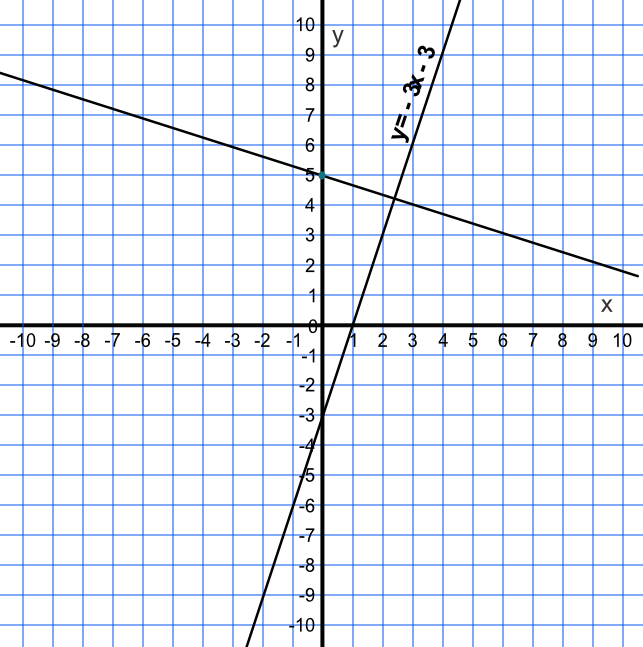Perpendicular Lines

## Perpendicular Lines

Perpendicular lines exist at right angles to each other.

Lines are perpendicular if the gradients of the two lines multiplied together = -1.

## Example 1

What is the equation of the line that is perpendicular to y = 3x -3 and also passes through (0, 5)?

The gradient of the original line is 3. The gradient of the perpendicular line = - frac(1)(3) (reciprocal of the original gradient multiplied by -1).

The line passes through (0, 5), which is the intercept value, c.Answer: y = -frac(1)(3)x + 5

## Example 2

Find the equation of the line perpendicular to y = frac(1)(2)x - 3 that passes through (4, 4).

The gradient of the second line is given by - 1 / frac(1)(2) = -2

The lines passes through the point (4, 4): 4 = -2 x 4 + c, where c is the intercept = 12

The equation of the line is y = -2x + 12

Answer: y = -2x + 12